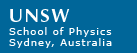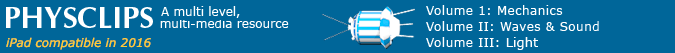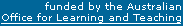How does pressure vary with volume change during adiabatic expansion and compression in an ideal gas? This page is an appendix to our discussion of sound. Air at atmospheric pressure is a nearly ideal gas, and sound transmission in air is close to adiabatic for normal frequencies and transmission distances. (We return to this assumption below.)

The first law of thermodynamics relates the change in internal energy dU to the work dW done by a system and the heat dQ added to it.

dU = dQ – dW

By definition, in an adiabatic process, the heat exchanged dQ = 0. Substituting this in the first law and rearranging gives

0 = dQ = dU + dW

The second term is easy: the work done dW when a system changes its volume V by dV is PdV.

The first term may be related to the specific heat, which is defined as the heat added per unit temperature change per mole of substance. If we add heat at constant volume, then the gas does not expand and so doesn't do work. So the added heat increases the internal energy U. So it follows that definition that the specific heat at constant volume is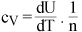, where n is the number of moles. Hence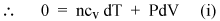Now the equation of state of an ideal gas is

nRT = PV (ii)

where R is the gas constant. Taking derivatives gives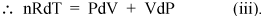We may now combine equations (i) and (iii) to eliminate T. (i) and (ii) give respectively these expressions for ncv dT:Collecting the PdV and VdP terms gives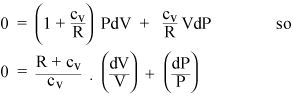Now if the gas is ideal, its internal energy is all kinetic, so the internal energy U depends only on T. From the equation of state of an ideal gas (ii), we can calculate the work dW done at constant pressure: it is just PdV = nRdT. So, if we add heat to a gas at constant pressure, we have to extra heat RdT for each mole of gas, beyond the heat we should have to add at constant volume. So the specific heat of an ideal gas at constant pressure is just cP  =  cv + R. The ratio of specific heats is given a standard symbol:. So we have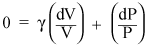(iv)

This is the main result that we need to discuss sound waves: we have just shown that the proportional change in pressure is – γ times the proportional change in volume or γ times the proportional change in density.

Let's not stop here, however, because we are only a few lines away from an important result. We know that d(ln x) = dx/x (see calculus for a proof). So we can write the preceding equation asand rearrange it to give(v)

Hence for an adiabatic process in an ideal gas,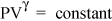.     (vi)

On reflection, you'll agree that cP > cV: At constant volume, all the heat you put in goes to increasing the internal energy and thus raising the temperature. At constant pressure, you need to put in not only the heat that raises the internal energy, but also an amount of heat equal to the work done when the system undergoes thermal expansion. For air, γ is about 1.4.

Of course, we may now substitute into (vi) from the equation of state (ii) to see how P and T or T and V are related for an adiabatic process.This work is licensed under a Creative Commons License.